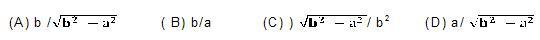# CBSE CLASS 10 MCQ TRIGONOMETRY1. If cos A = 4/5 , then the value of tan A is(A) 3/5 (B)3/4 (C)4/3 (D)5/32. If sin A = 1/2 , then the value of cot A is(A) 3 (B) 1/3 (C) 3/2 (D) 13. The value of the expression [cosec (75 + q) sec (15 q tan (55 + q+ cot (35 q)] is(A) 1 (B) 0 (C) 1 (D) 3/24. Given that sinq= a/b , then cosq is equal to5. If cos (a + b) = 0, then sin (a - b) can be reduced to(A) cos b (B) cos 2b (C) sin α (D) sin 2a6. The value of (tan1 tan 2 tan3 ... tan 89) is(A) 0 (B) 1 (C) 2 (D)1 / 27. If cos 9a= sinα and 9a a is(A) 1/√3 (B) √ 3 (C) 1 (D) 08. If DABC is right angled at C, then the value of cos (A+B) is(A) 0 (B) 1 (C) 1/2 (D)√3/29. If sinA + sin2A = 1, then the value of the expression (cos2A + cos4A) is(A) 1 (B) 1/2 (C) 2 (D) 310. Given that sina= 1/2 and cosb =1/2 , then the value of (a + b) is(A) 0 (B) 30 (C) 60 (D) 9011. The value of the expression [sin2220sin2680/ cos2220cos2680+ sin2630cos630sin 270] is (A) 3 (B) 2 (C) 1 (D) 012. If 4 tanq = 3, then [4sinq - cosq ] / [4sinq + cos q ] is equal to(A) 2/3 (B) 1/3 (C) 1/2 (D) 3/413. If sinq cosq = 0, then the value of (sin4q + cos4qθ) is(A) 1 (B) 3/4 (C) 1/2 (D) 1/414. sin (45 + q) cos (45 q) is equal to(A) 2cosq (B) 0 (C) 2 sin q (D) 115. A pole 6 m high casts a shadow 2 √3m long on theground, then the Suns elevation is(A ) 60 (B) 45 (C) 30 (D) 90

Due to time constraint we cannot solve the entire question paper/ rest of your queries. But, we can help you in the questions which you think are really difficult. Please mention the same and go through our study material and revision notes to solve the remaining ones on your own.

• -8
What are you looking for?